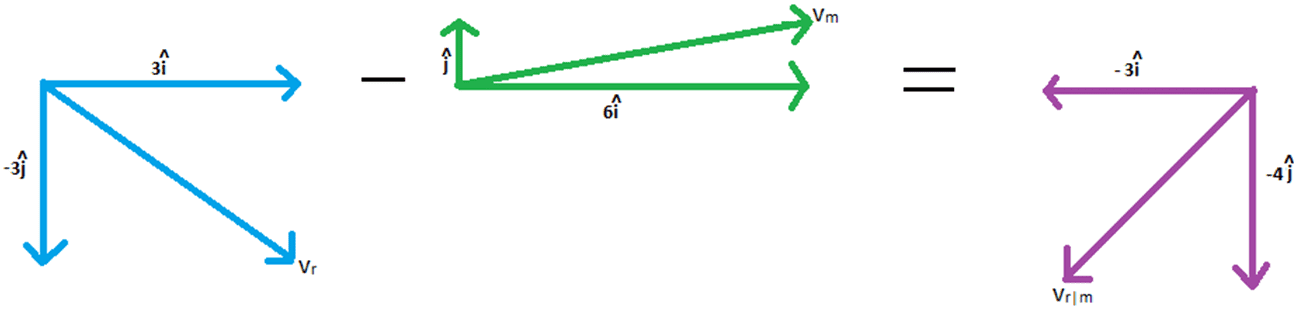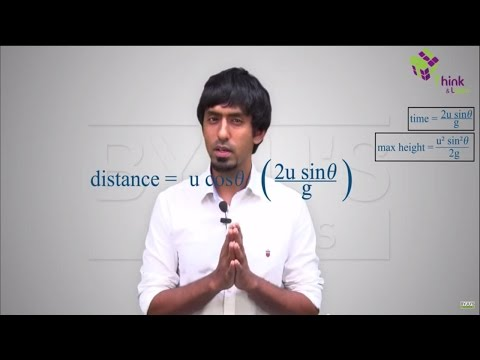# Motion In A Plane

## Introduction To Motion In Two Dimensions

We have learned about motion in a straight line and the three equations of motion. Now we will learn about motion in a plane. When we are talking about motion in a plane, we are talking about motion in two dimensions, since two dimensions make a plane. So we are taking two axes into consideration, generally X-axis and Y-axis. In order to derive equations of motion, we need to know about motion in one dimension.

Study the motion in a plane with the help of topics like vectors, projectile motion, relative motion and more.

The equations of motion in a straight line are:

 $$\begin{array}{l}v=u+at\end{array}$$ $$\begin{array}{l}s=ut+\frac{1}{2}at^{2}\end{array}$$ $$\begin{array}{l}v^2=u^2+2as\end{array}$$

Where,

• v = final velocity of the particle
• u = initial velocity of the particle
• s = displacement of the particle
• a = acceleration of the particle
• t = time interval in which the particle is in consideration

In a plane, we have to apply the same equations separately in both the directions: Y axis and Y-axis.

This would give us the equations for motion in a plane:

 $$\begin{array}{l}v_y=u_y+a_yt\end{array}$$ $$\begin{array}{l}s_y=u_yt+\frac{1}{2}a_yt^{2}\end{array}$$ $$\begin{array}{l}v_y^2=u_y^2+2a_ys\end{array}$$

Where,

• vy= final velocity of the particle in y direction
• uy= initial velocity of the particle in y direction
• sy= displacement of the particle in y direction
• ay= acceleration of the particle in y direction
• t = time interval in which the particle is in consideration

Similarly, for X-axis:

 $$\begin{array}{l}V_x=u_x+a_xt\end{array}$$ $$\begin{array}{l}S_x=u_xt+\frac{1}{2}a_x t^{2}\end{array}$$ $$\begin{array}{l}V_x^2=u_x^2+2a_x s\end{array}$$

Where,

• vx= final velocity of the particle in x direction
• ux= initial velocity of the particle in x direction
• sx= displacement of the particle in x direction
• ax= acceleration of the particle in x direction
• t = time interval in which the particle is in consideration

## Relative Motion

When we say the motion of body A relative to B we mean motion of A, as observed from B’s frame of reference. Mathematically it is represented as:

 $$\begin{array}{l}V_{BA}~=~V_B~–~V_A\end{array}$$

Where,

• VBA = Velocity of B as observed from A.
• VB = Velocity of B from the earth as the reference frame.
• VA = Velocity of A from the earth as the reference frame.

Watch this video to have a better and visual understanding of motion in a plane.Consider a man running uphill while it is raining. It is difficult to decide from which side the man will get wet if we do not know the relative m of the man and rain with respect to each other. Suppose, the components of velocity of man are given as 6i + j and that of rain is given by 3i – 3j. If we can find the angle at which the rain hits the man, we can easily decide from which direction the man gets hit by the rain. To find the relative velocity or relative motion of rain with respect to man, the velocity of a man with respect to rain must be subtracted, i.e.,

 $$\begin{array}{l}\overrightarrow{v_{r|m}}~ =~\overrightarrow{v_{r}} ~-~\overrightarrow{v_{m}}\end{array}$$

Relative motion can be explained using the vector representation as:It can be seen that with respect to the man, the rain is falling at an angle of

$$\begin{array}{l}tan^{-1} \left( \frac 34 \right)\end{array}$$
. So, if the man is running in positive X- direction then rain must hit him from the front direction. So, if the man has to wear a bag, he must wear it on his back to prevent it from rain. Thus only by knowing the velocities, the direction of hitting of rain can be determined. Now, what happens if the horizontal component of the velocity of rain and man becomes equal? Interesting, isn’t it? Well, in such a situation the rain will not hit the man from either side. So, to answer the question of whether one should run fast or slow in the rain to get less wet, it all depends on the velocities or relative motion.

#### Relative motion of rain with respect to the moving body (man).#### Top 15 Most Important and Expected Questions – Motion in a Plane.s

## Projectile Motion

Projectile motion is one of the most common examples of motion in a plane. In projectile motion, the only acceleration acting is in the vertical direction, which is acceleration due to gravity (g). Therefore, equations of motion can be applied separately in X-axis and Y-axis to find the unknown parameters. To know more about projectile motion, watch the video give below:Hope you have got a brief insight of motion in a plane of motion in two dimensions. Learn more about other related concepts of physics and their applications, with interactive videos by visiting BYJU’S.

## Frequently Asked Questions on Motion In A Plane

### What is motion in a plane?

Motion in a plane means motion in a two-dimensional plane which includes x-axis and y-axis.

### What is one-dimensional motion and two-dimensional motion?

When the object travels in a straight line, irrespective of the direction is known as one-dimensional motion. When the object travels in x and y coordinates with a constant velocity, it is known as two-dimensional motion.

### What is accelerated motion?

When the velocity is increased gradually with respect to the time, it is known as accelerated motion.

### What is oscillatory motion?

The to and fro motion of the object about its mean position is known as oscillatory motion.

### What is projectile motion?

Projectile motion is when the object is projected into air and gravity is the only force acting upon the object.

Test your Knowledge on motion in a plane

#### 1 Comment

1. extra ordinary work is done by you